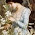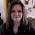### Chocolate Math

A very dear friend of mine sent me this fun little "math joke".

DON'T CHEAT BY SCROLLING DOWN FIRST!
It takes less than a minute .
Work this out as you read .
Be sure you don't read the bottom until you've worked it out!
This is not one of those waste of time things, it's fun.

1. First of all, pick the number of times a week that you would like to have chocolate (more than once but less than 10)
2. Multiply this number by 2 (just to be bold)
4. Multiply it by 50 -- I'll wait while you get the calculator

6. Now subtract the four digit year that you were born.
You should have a three digit number
The first digit of this was your original number
(i.e., how many times you want to have chocolate each week).
The next two numbers are

RosieBear asked me how does it work? Or rather why does it work. So I did some internet searching and discovered that it is an algebraic formula and it is really simple. Ever want to know how old someone is but you only know their birthdate? How would you find their current age? You'd take the current year and subtract the year of their birth. If they haven't had their birthday yet, you'd subtract one year. So since honeybear was born in 1971 and hasn't had his birthday yet I'd do this: 2010-1971=39 but he hasn't had his birthday yet so 39-1=38. He's 38 years old. I do this frequently for trying to remember how old my Grandparents would be if still living or a couple of weeks ago to verify how old my dad would have been on his birthday. 2010-1936= 74. My dad would be 74 right now if he were still alive.

Now you may be saying.. but Tess... it doesn't ask me what year it is and it's having me multiply by 2 then add 5 and then multiply 50 and then add some strange number.

Ah! But *it* has taken the current year into consideration! The formula that I found on the internet ( How does "Chocolate Math" work is just one of the several sites I found) in algebraic form has the year "hidden"!!!!

50(2x + 5) + 1759 {or 1760 if you have had your birthday} - [year of birth]. If you distribute the 50 you get 100X +250. The 100X puts you choice for chocolate in the hundreds column. 250 +1759 = 2009 (250+1760= 2010)
So basically you really have this: 100x + last year if you haven't had your birthday or this year if you have - the year you were born. 1759 for no birthday yet and 1760 for already had birthday will only work for this year (2010) however the Age Calculator *will* work for any year you want it to so long as you remember this trick:
Current Year - 250 = number to add in step 5 if you have already had your birthday. Current Year- 251= number to add in step 5 if you have NOT had your birthday. I found sources for this "Chocolate Math" from 2004, 2006, 2007, 2008 and 2009. Exact wording, too. The only change was the number you add in step 5.

Rosiebear learned that math really can be fun. It can be tasty too if you actually get to eat the chocolate!

1.Mine came out with -168, and the original number was 4. Maybe I'll try it again...

~Carrie

2.Okay, the problem was that I multiplied by 5 in step 4, rather than 50! No wonder... :)

~Carrie

3.It's fun isn't it? (When it works). Hope your family is well!

4.